# `skimage.exposure`#

 `skimage.exposure.adjust_gamma` Performs Gamma Correction on the input image. `skimage.exposure.adjust_log` Performs Logarithmic correction on the input image. `skimage.exposure.adjust_sigmoid` Performs Sigmoid Correction on the input image. `skimage.exposure.cumulative_distribution` Return cumulative distribution function (cdf) for the given image. `skimage.exposure.equalize_adapthist` Contrast Limited Adaptive Histogram Equalization (CLAHE). `skimage.exposure.equalize_hist` Return image after histogram equalization. `skimage.exposure.histogram` Return histogram of image. `skimage.exposure.is_low_contrast` Determine if an image is low contrast. `skimage.exposure.match_histograms` Adjust an image so that its cumulative histogram matches that of another. `skimage.exposure.rescale_intensity` Return image after stretching or shrinking its intensity levels.

Performs Gamma Correction on the input image.

Also known as Power Law Transform. This function transforms the input image pixelwise according to the equation `O = I**gamma` after scaling each pixel to the range 0 to 1.

Parameters:
imagendarray

Input image.

gammafloat, optional

Non negative real number. Default value is 1.

gainfloat, optional

The constant multiplier. Default value is 1.

Returns:
outndarray

Gamma corrected output image.

Notes

For gamma greater than 1, the histogram will shift towards left and the output image will be darker than the input image.

For gamma less than 1, the histogram will shift towards right and the output image will be brighter than the input image.

References

Examples

```>>> from skimage import data, exposure, img_as_float
>>> image = img_as_float(data.moon())
>>> # Output is darker for gamma > 1
>>> image.mean() > gamma_corrected.mean()
True
```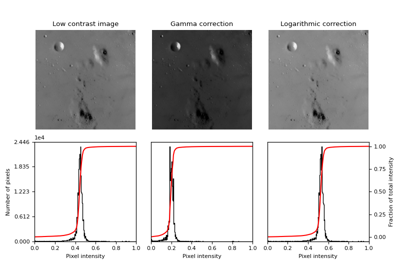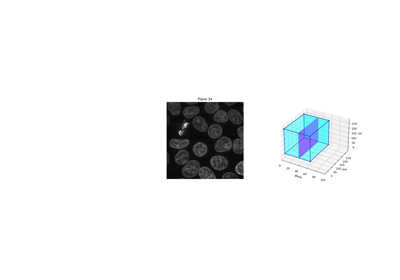Explore 3D images (of cells)

Explore 3D images (of cells)

Performs Logarithmic correction on the input image.

This function transforms the input image pixelwise according to the equation `O = gain*log(1 + I)` after scaling each pixel to the range 0 to 1. For inverse logarithmic correction, the equation is `O = gain*(2**I - 1)`.

Parameters:
imagendarray

Input image.

gainfloat, optional

The constant multiplier. Default value is 1.

invfloat, optional

If True, it performs inverse logarithmic correction, else correction will be logarithmic. Defaults to False.

Returns:
outndarray

Logarithm corrected output image.

ReferencesPerforms Sigmoid Correction on the input image.

Also known as Contrast Adjustment. This function transforms the input image pixelwise according to the equation `O = 1/(1 + exp*(gain*(cutoff - I)))` after scaling each pixel to the range 0 to 1.

Parameters:
imagendarray

Input image.

cutofffloat, optional

Cutoff of the sigmoid function that shifts the characteristic curve in horizontal direction. Default value is 0.5.

gainfloat, optional

The constant multiplier in exponential’s power of sigmoid function. Default value is 10.

invbool, optional

If True, returns the negative sigmoid correction. Defaults to False.

Returns:
outndarray

Sigmoid corrected output image.

References



Gustav J. Braun, “Image Lightness Rescaling Using Sigmoidal Contrast Enhancement Functions”, http://markfairchild.org/PDFs/PAP07.pdf

skimage.exposure.cumulative_distribution(image, nbins=256)[source]#

Return cumulative distribution function (cdf) for the given image.

Parameters:
imagearray

Image array.

nbinsint, optional

Number of bins for image histogram.

Returns:
img_cdfarray

Values of cumulative distribution function.

bin_centersarray

Centers of bins.

References

Examples

```>>> from skimage import data, exposure, img_as_float
>>> image = img_as_float(data.camera())
>>> hi = exposure.histogram(image)
>>> cdf = exposure.cumulative_distribution(image)
>>> all(cdf == np.cumsum(hi)/float(image.size))
True
```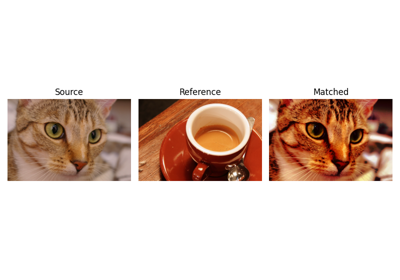Histogram matching

Histogram matching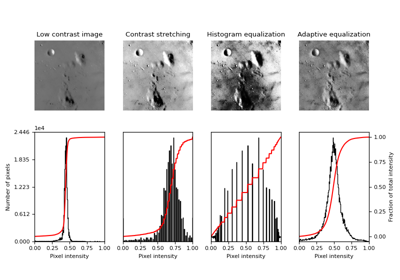Histogram Equalization

Histogram Equalization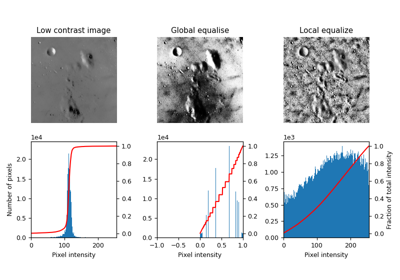Local Histogram Equalization

Local Histogram EqualizationExplore 3D images (of cells)

Explore 3D images (of cells)

Contrast Limited Adaptive Histogram Equalization (CLAHE).

An algorithm for local contrast enhancement, that uses histograms computed over different tile regions of the image. Local details can therefore be enhanced even in regions that are darker or lighter than most of the image.

Parameters:
image(N1, …,NN[, C]) ndarray

Input image.

kernel_sizeint or array_like, optional

Defines the shape of contextual regions used in the algorithm. If iterable is passed, it must have the same number of elements as `image.ndim` (without color channel). If integer, it is broadcasted to each image dimension. By default, `kernel_size` is 1/8 of `image` height by 1/8 of its width.

clip_limitfloat, optional

Clipping limit, normalized between 0 and 1 (higher values give more contrast).

nbinsint, optional

Number of gray bins for histogram (“data range”).

Returns:
out(N1, …,NN[, C]) ndarray

Equalized image with float64 dtype.

Notes

• For color images, the following steps are performed:
• The image is converted to HSV color space

• The CLAHE algorithm is run on the V (Value) channel

• The image is converted back to RGB space and returned

• For RGBA images, the original alpha channel is removed.

Changed in version 0.17: The values returned by this function are slightly shifted upwards because of an internal change in rounding behavior.

ReferencesHistogram Equalization

Histogram Equalization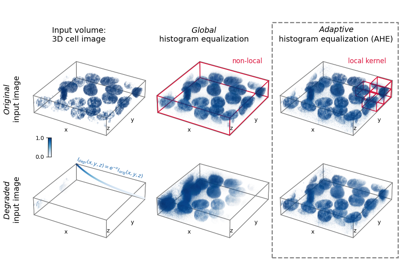Return image after histogram equalization.

Parameters:
imagearray

Image array.

nbinsint, optional

Number of bins for image histogram. Note: this argument is ignored for integer images, for which each integer is its own bin.

maskndarray of bools or 0s and 1s, optional

Array of same shape as image. Only points at which mask == True are used for the equalization, which is applied to the whole image.

Returns:
outfloat array

Image array after histogram equalization.

Notes

This function is adapted from  with the author’s permission.

ReferencesHistogram Equalization

Histogram EqualizationLocal Histogram Equalization

Local Histogram Equalization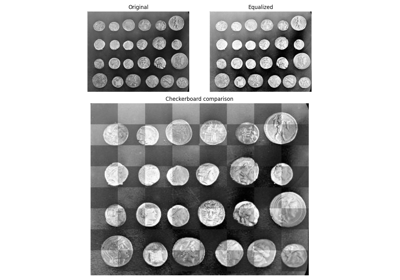Visual image comparison

Visual image comparisonExplore 3D images (of cells)

Explore 3D images (of cells)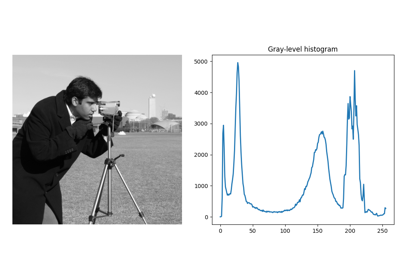Rank filters

Rank filters

skimage.exposure.histogram(image, nbins=256, source_range='image', normalize=False, *, channel_axis=None)[source]#

Return histogram of image.

Unlike `numpy.histogram`, this function returns the centers of bins and does not rebin integer arrays. For integer arrays, each integer value has its own bin, which improves speed and intensity-resolution.

If channel_axis is not set, the histogram is computed on the flattened image. For color or multichannel images, set `channel_axis` to use a common binning for all channels. Alternatively, one may apply the function separately on each channel to obtain a histogram for each color channel with separate binning.

Parameters:
imagearray

Input image.

nbinsint, optional

Number of bins used to calculate histogram. This value is ignored for integer arrays.

source_rangestring, optional

‘image’ (default) determines the range from the input image. ‘dtype’ determines the range from the expected range of the images of that data type.

normalizebool, optional

If True, normalize the histogram by the sum of its values.

channel_axisint or None, optional

If None, the image is assumed to be a grayscale (single channel) image. Otherwise, this parameter indicates which axis of the array corresponds to channels.

Returns:
histarray

The values of the histogram. When `channel_axis` is not None, hist will be a 2D array where the first axis corresponds to channels.

bin_centersarray

The values at the center of the bins.

Examples

```>>> from skimage import data, exposure, img_as_float
>>> image = img_as_float(data.camera())
>>> np.histogram(image, bins=2)
(array([ 93585, 168559]), array([0. , 0.5, 1. ]))
>>> exposure.histogram(image, nbins=2)
(array([ 93585, 168559]), array([0.25, 0.75]))
```Histogram matching

Histogram matching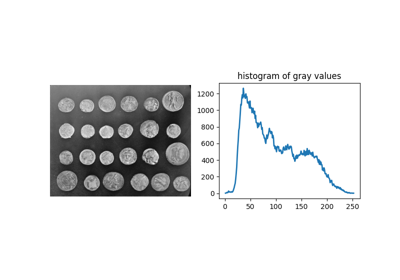Comparing edge-based and region-based segmentation

Comparing edge-based and region-based segmentationRank filters

Rank filters

skimage.exposure.is_low_contrast(image, fraction_threshold=0.05, lower_percentile=1, upper_percentile=99, method='linear')[source]#

Determine if an image is low contrast.

Parameters:
imagearray-like

The image under test.

fraction_thresholdfloat, optional

The low contrast fraction threshold. An image is considered low- contrast when its range of brightness spans less than this fraction of its data type’s full range. 

lower_percentilefloat, optional

Disregard values below this percentile when computing image contrast.

upper_percentilefloat, optional

Disregard values above this percentile when computing image contrast.

methodstr, optional

The contrast determination method. Right now the only available option is “linear”.

Returns:
outbool

True when the image is determined to be low contrast.

Notes

For boolean images, this function returns False only if all values are the same (the method, threshold, and percentile arguments are ignored).

References

Examples

```>>> image = np.linspace(0, 0.04, 100)
>>> is_low_contrast(image)
True
>>> image[-1] = 1
>>> is_low_contrast(image)
True
>>> is_low_contrast(image, upper_percentile=100)
False
```

skimage.exposure.match_histograms(image, reference, *, channel_axis=None)[source]#

Adjust an image so that its cumulative histogram matches that of another.

The adjustment is applied separately for each channel.

Parameters:
imagendarray

Input image. Can be gray-scale or in color.

referencendarray

Image to match histogram of. Must have the same number of channels as image.

channel_axisint or None, optional

If None, the image is assumed to be a grayscale (single channel) image. Otherwise, this parameter indicates which axis of the array corresponds to channels.

Returns:
matchedndarray

Transformed input image.

Raises:
ValueError

Thrown when the number of channels in the input image and the reference differ.

ReferencesHistogram matching

Histogram matching

skimage.exposure.rescale_intensity(image, in_range='image', out_range='dtype')[source]#

Return image after stretching or shrinking its intensity levels.

The desired intensity range of the input and output, in_range and out_range respectively, are used to stretch or shrink the intensity range of the input image. See examples below.

Parameters:
imagearray

Image array.

in_range, out_rangestr or 2-tuple, optional

Min and max intensity values of input and output image. The possible values for this parameter are enumerated below.

‘image’

Use image min/max as the intensity range.

‘dtype’

Use min/max of the image’s dtype as the intensity range.

dtype-name

Use intensity range based on desired dtype. Must be valid key in DTYPE_RANGE.

2-tuple

Use range_values as explicit min/max intensities.

Returns:
outarray

Image array after rescaling its intensity. This image is the same dtype as the input image.

Notes

Changed in version 0.17: The dtype of the output array has changed to match the input dtype, or float if the output range is specified by a pair of values.

Examples

By default, the min/max intensities of the input image are stretched to the limits allowed by the image’s dtype, since in_range defaults to ‘image’ and out_range defaults to ‘dtype’:

```>>> image = np.array([51, 102, 153], dtype=np.uint8)
>>> rescale_intensity(image)
array([  0, 127, 255], dtype=uint8)
```

It’s easy to accidentally convert an image dtype from uint8 to float:

```>>> 1.0 * image
array([ 51., 102., 153.])
```

Use `rescale_intensity` to rescale to the proper range for float dtypes:

```>>> image_float = 1.0 * image
>>> rescale_intensity(image_float)
array([0. , 0.5, 1. ])
```

To maintain the low contrast of the original, use the in_range parameter:

```>>> rescale_intensity(image_float, in_range=(0, 255))
array([0.2, 0.4, 0.6])
```

If the min/max value of in_range is more/less than the min/max image intensity, then the intensity levels are clipped:

```>>> rescale_intensity(image_float, in_range=(0, 102))
array([0.5, 1. , 1. ])
```

If you have an image with signed integers but want to rescale the image to just the positive range, use the out_range parameter. In that case, the output dtype will be float:

```>>> image = np.array([-10, 0, 10], dtype=np.int8)
>>> rescale_intensity(image, out_range=(0, 127))
array([  0. ,  63.5, 127. ])
```

To get the desired range with a specific dtype, use `.astype()`:

```>>> rescale_intensity(image, out_range=(0, 127)).astype(np.int8)
array([  0,  63, 127], dtype=int8)
```

If the input image is constant, the output will be clipped directly to the output range: >>> image = np.array([130, 130, 130], dtype=np.int32) >>> rescale_intensity(image, out_range=(0, 127)).astype(np.int32) array([127, 127, 127], dtype=int32)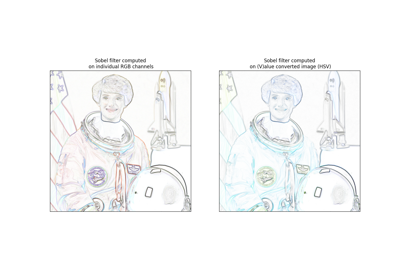Adapting gray-scale filters to RGB images

Adapting gray-scale filters to RGB images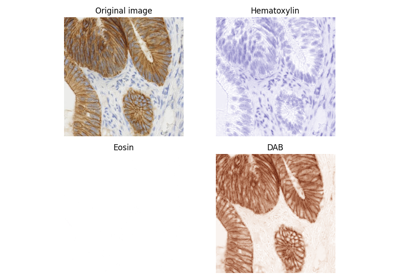Separate colors in immunohistochemical staining

Separate colors in immunohistochemical stainingHistogram Equalization

Histogram Equalization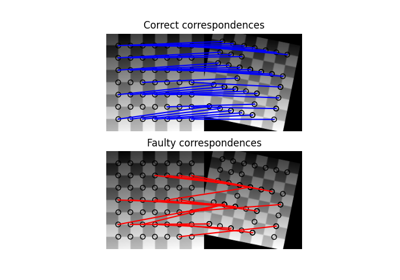Robust matching using RANSAC

Robust matching using RANSAC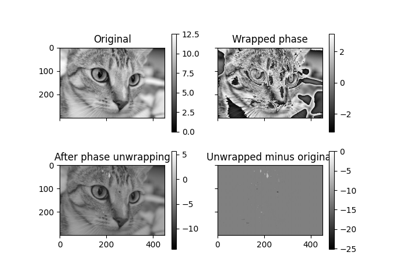Phase Unwrapping

Phase Unwrapping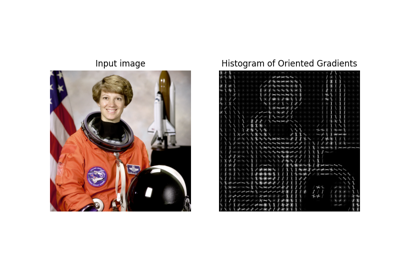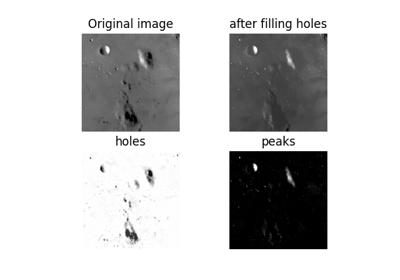Filling holes and finding peaks

Filling holes and finding peaks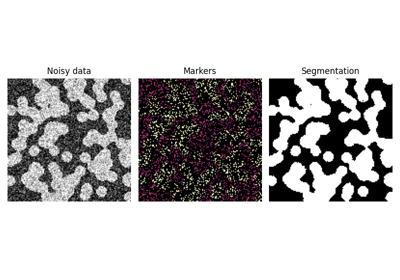Random walker segmentation

Random walker segmentation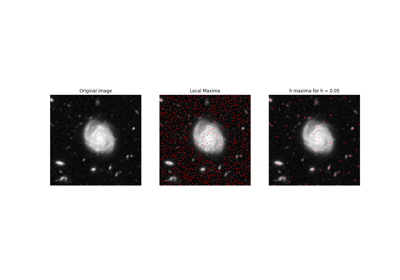Extrema

ExtremaExplore 3D images (of cells)

Explore 3D images (of cells)Rank filters

Rank filters